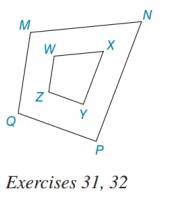Chapter 5.1, Problem 31EElementary Geometry For College St...

7th Edition
Alexander + 2 others
ISBN: 9781337614085

Solutions

Chapter
SectionElementary Geometry For College St...

7th Edition
Alexander + 2 others
ISBN: 9781337614085
Textbook Problem

For the quadrilaterals shown, M N W X = N P X Y = P Q Y Z = M Q W Z . If MN = 7, WX = 3, and PQ = 6, to find YZ.To determine

To find:

The value of YZ.

Explanation

According to Means-Extremes Property, in a proportion, the product of the means equals the product of the extremes.

Calculation:

It is given that MNWX=NPXY=PQYZ=MQWZ, MN = 7, WX = 3 and PQ = 6

Consider, MNWX=PQYZ

Substitute MN = 7, WX = 3 and PQ = 6 in MNWX=PQYZ

Still sussing out bartleby?

Check out a sample textbook solution.

See a sample solution

The Solution to Your Study Problems

Bartleby provides explanations to thousands of textbook problems written by our experts, many with advanced degrees!

Get Started

Find more solutions based on key concepts